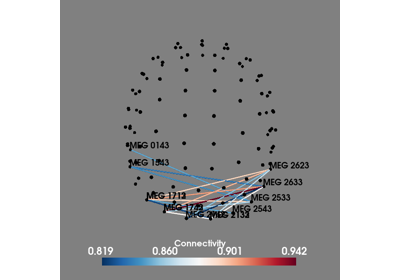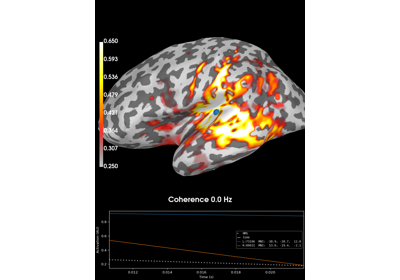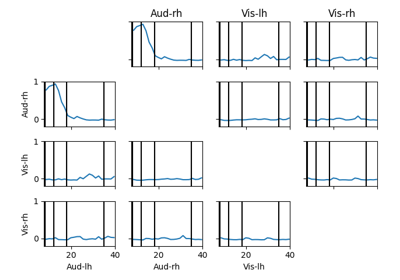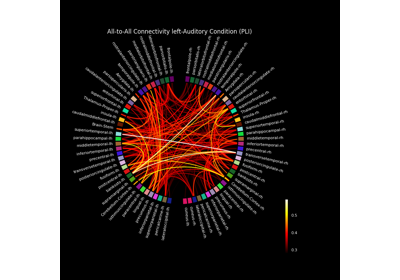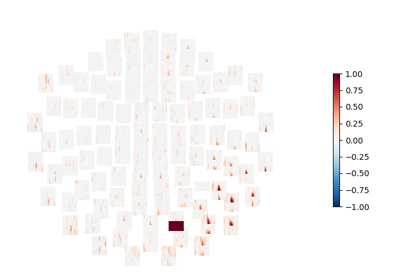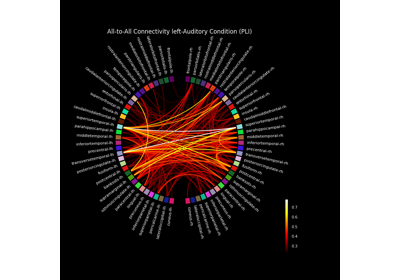# mne_connectivity.spectral_connectivity¶

mne_connectivity.spectral_connectivity(data, names=None, method='coh', indices=None, sfreq=6.283185307179586, mode='multitaper', fmin=None, fmax=inf, fskip=0, faverage=False, tmin=None, tmax=None, mt_bandwidth=None, mt_adaptive=False, mt_low_bias=True, cwt_freqs=None, cwt_n_cycles=7, block_size=1000, n_jobs=1, verbose=None)[source]

Compute frequency- and time-frequency-domain connectivity measures.

The connectivity method(s) are specified using the “method” parameter. All methods are based on estimates of the cross- and power spectral densities (CSD/PSD) Sxy and Sxx, Syy.

Parameters

data : array-like, shape=(n_epochs, n_signals, n_times) | Epochs

The data from which to compute connectivity. Note that it is also possible to combine multiple signals by providing a list of tuples, e.g., data = [(arr_0, stc_0), (arr_1, stc_1), (arr_2, stc_2)], corresponds to 3 epochs, and arr_* could be an array with the same number of time points as stc_*. The array-like object can also be a list/generator of array, shape =(n_signals, n_times), or a list/generator of SourceEstimate or VolSourceEstimate objects.

method : str | list of str

Connectivity measure(s) to compute. These can be ```['coh', 'cohy', 'imcoh', 'plv', 'ciplv', 'ppc', 'pli', 'wpli', 'wpli2_debiased']```.

indices : tuple of array | None

Two arrays with indices of connections for which to compute connectivity. If None, all connections are computed.

sfreq : float

The sampling frequency.

mode : str

Spectrum estimation mode can be either: ‘multitaper’, ‘fourier’, or ‘cwt_morlet’.

fmin : float | tuple of float

The lower frequency of interest. Multiple bands are defined using a tuple, e.g., (8., 20.) for two bands with 8Hz and 20Hz lower freq. If None the frequency corresponding to an epoch length of 5 cycles is used.

fmax : float | tuple of float

The upper frequency of interest. Multiple bands are dedined using a tuple, e.g. (13., 30.) for two band with 13Hz and 30Hz upper freq.

fskip : int

Omit every “(fskip + 1)-th” frequency bin to decimate in frequency domain.

faverage : bool

Average connectivity scores for each frequency band. If True, the output freqs will be a list with arrays of the frequencies that were averaged.

tmin : float | None

Time to start connectivity estimation. Note: when “data” is an array, the first sample is assumed to be at time 0. For other types (Epochs, etc.), the time information contained in the object is used to compute the time indices.

tmax : float | None

Time to end connectivity estimation. Note: when “data” is an array, the first sample is assumed to be at time 0. For other types (Epochs, etc.), the time information contained in the object is used to compute the time indices.

mt_bandwidth : float | None

The bandwidth of the multitaper windowing function in Hz. Only used in ‘multitaper’ mode.

Use adaptive weights to combine the tapered spectra into PSD. Only used in ‘multitaper’ mode.

mt_low_bias : bool

Only use tapers with more than 90% spectral concentration within bandwidth. Only used in ‘multitaper’ mode.

cwt_freqs : array

Array of frequencies of interest. Only used in ‘cwt_morlet’ mode.

cwt_n_cycles : float | array of float

Number of cycles. Fixed number or one per frequency. Only used in ‘cwt_morlet’ mode.

block_size : int

How many connections to compute at once (higher numbers are faster but require more memory).

n_jobs : int

How many epochs to process in parallel.

verbose : bool | str | int | None

If not None, override default verbose level (see `mne.verbose()` and Logging documentation for more). If used, it should be passed as a keyword-argument only.

Returns

con : array | list of array

Computed connectivity measure(s). Either an instance of `SpectralConnectivity` or `SpectroTemporalConnectivity`. The shape of each connectivity dataset is either (n_signals ** 2, n_freqs) mode: ‘multitaper’ or ‘fourier’ (n_signals ** 2, n_freqs, n_times) mode: ‘cwt_morlet’ when “indices” is None, or (n_con, n_freqs) mode: ‘multitaper’ or ‘fourier’ (n_con, n_freqs, n_times) mode: ‘cwt_morlet’ when “indices” is specified and “n_con = len(indices)”.

Notes

Please note that the interpretation of the measures in this function depends on the data and underlying assumptions and does not necessarily reflect a causal relationship between brain regions.

These measures are not to be interpreted over time. Each Epoch passed into the dataset is interpreted as an independent sample of the same connectivity structure. Within each Epoch, it is assumed that the spectral measure is stationary. The spectral measures implemented in this function are computed across Epochs. Thus, spectral measures computed with only one Epoch will result in errorful values.

The spectral densities can be estimated using a multitaper method with digital prolate spheroidal sequence (DPSS) windows, a discrete Fourier transform with Hanning windows, or a continuous wavelet transform using Morlet wavelets. The spectral estimation mode is specified using the “mode” parameter.

By default, the connectivity between all signals is computed (only connections corresponding to the lower-triangular part of the connectivity matrix). If one is only interested in the connectivity between some signals, the “indices” parameter can be used. For example, to compute the connectivity between the signal with index 0 and signals “2, 3, 4” (a total of 3 connections) one can use the following:

```indices = (np.array([0, 0, 0]),    # row indices
np.array([2, 3, 4]))    # col indices

con_flat = spectral_connectivity(data, method='coh',
indices=indices, ...)
```

In this case con_flat.shape = (3, n_freqs). The connectivity scores are in the same order as defined indices.

Supported Connectivity Measures

The connectivity method(s) is specified using the “method” parameter. The following methods are supported (note: `E[]` denotes average over epochs). Multiple measures can be computed at once by using a list/tuple, e.g., `['coh', 'pli']` to compute coherence and PLI.

‘coh’ : Coherence given by:

```         | E[Sxy] |
C = ---------------------
sqrt(E[Sxx] * E[Syy])
```

‘cohy’ : Coherency given by:

```           E[Sxy]
C = ---------------------
sqrt(E[Sxx] * E[Syy])
```

‘imcoh’ : Imaginary coherence 1 given by:

```          Im(E[Sxy])
C = ----------------------
sqrt(E[Sxx] * E[Syy])
```

‘plv’ : Phase-Locking Value (PLV) 2 given by:

```PLV = |E[Sxy/|Sxy|]|
```

‘ciplv’ : corrected imaginary PLV (icPLV) 3 given by:

```                 |E[Im(Sxy/|Sxy|)]|
ciPLV = ------------------------------------
sqrt(1 - |E[real(Sxy/|Sxy|)]| ** 2)
```

‘ppc’ : Pairwise Phase Consistency (PPC), an unbiased estimator of squared PLV 4.

‘pli’ : Phase Lag Index (PLI) 5 given by:

```PLI = |E[sign(Im(Sxy))]|
```

‘pli2_unbiased’ : Unbiased estimator of squared PLI 6.

‘wpli’ : Weighted Phase Lag Index (WPLI) 6 given by:

```          |E[Im(Sxy)]|
WPLI = ------------------
E[|Im(Sxy)|]
```

‘wpli2_debiased’ : Debiased estimator of squared WPLI 6.

References

1

Guido Nolte, Ou Bai, Lewis Wheaton, Zoltan Mari, Sherry Vorbach, and Mark Hallett. Identifying true brain interaction from EEG data using the imaginary part of coherency. Clinical Neurophysiology, 115(10):2292–2307, 2004. doi:10.1016/j.clinph.2004.04.029.

2

Jean-Philippe Lachaux, Eugenio Rodriguez, Jacques Martinerie, and Francisco J. Varela. Measuring phase synchrony in brain signals. Human Brain Mapping, 8(4):194–208, 1999. doi:10.1002/(SICI)1097-0193(1999)8:4<194::AID-HBM4>3.0.CO;2-C.

3

Ernesto Pereda Ricardo Bruña, Fernando Maestú. Phase locking value revisited: teaching new tricks to an old dog. Journal of Neural Engineering, 15(5):056011, 2018. doi:10.1088/1741-2552/aacfe4.

4

Martin Vinck, Marijn van Wingerden, Thilo Womelsdorf, Pascal Fries, and Cyriel M.A. Pennartz. The pairwise phase consistency: a bias-free measure of rhythmic neuronal synchronization. NeuroImage, 51(1):112–122, 2010. doi:10.1016/j.neuroimage.2010.01.073.

5

Cornelis J. Stam, Guido Nolte, and Andreas Daffertshofer. Phase lag index: assessment of functional connectivity from multi channel EEG and MEG with diminished bias from common sources. Human Brain Mapping, 28(11):1178–1193, 2007. doi:10.1002/hbm.20346.

6(1,2,3)

Martin Vinck, Robert Oostenveld, Marijn van Wingerden, Franscesco Battaglia, and Cyriel M.A. Pennartz. An improved index of phase-synchronization for electrophysiological data in the presence of volume-conduction, noise and sample-size bias. NeuroImage, 55(4):1548–1565, 2011. doi:10.1016/j.neuroimage.2011.01.055.

## Examples using `mne_connectivity.spectral_connectivity`¶